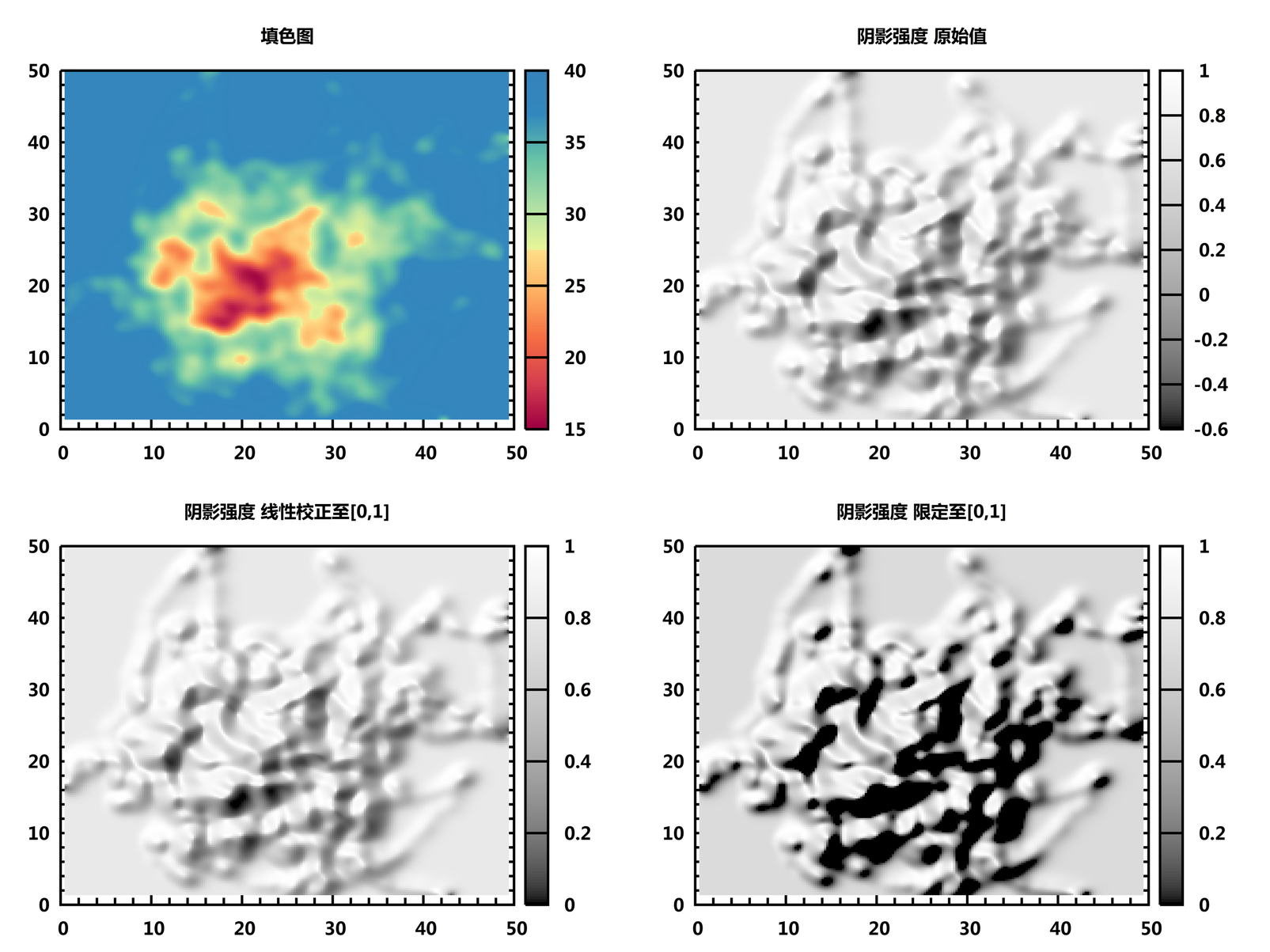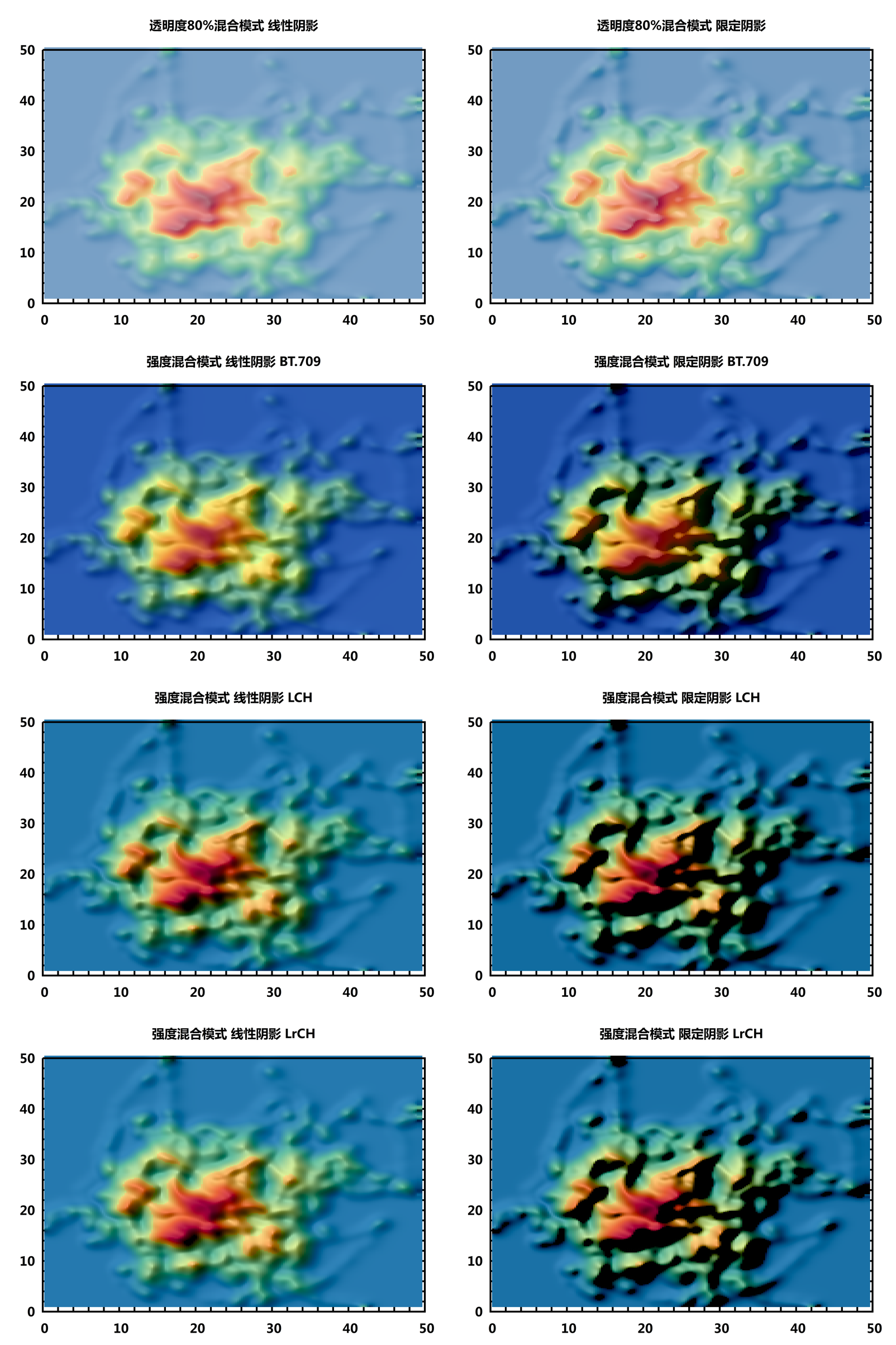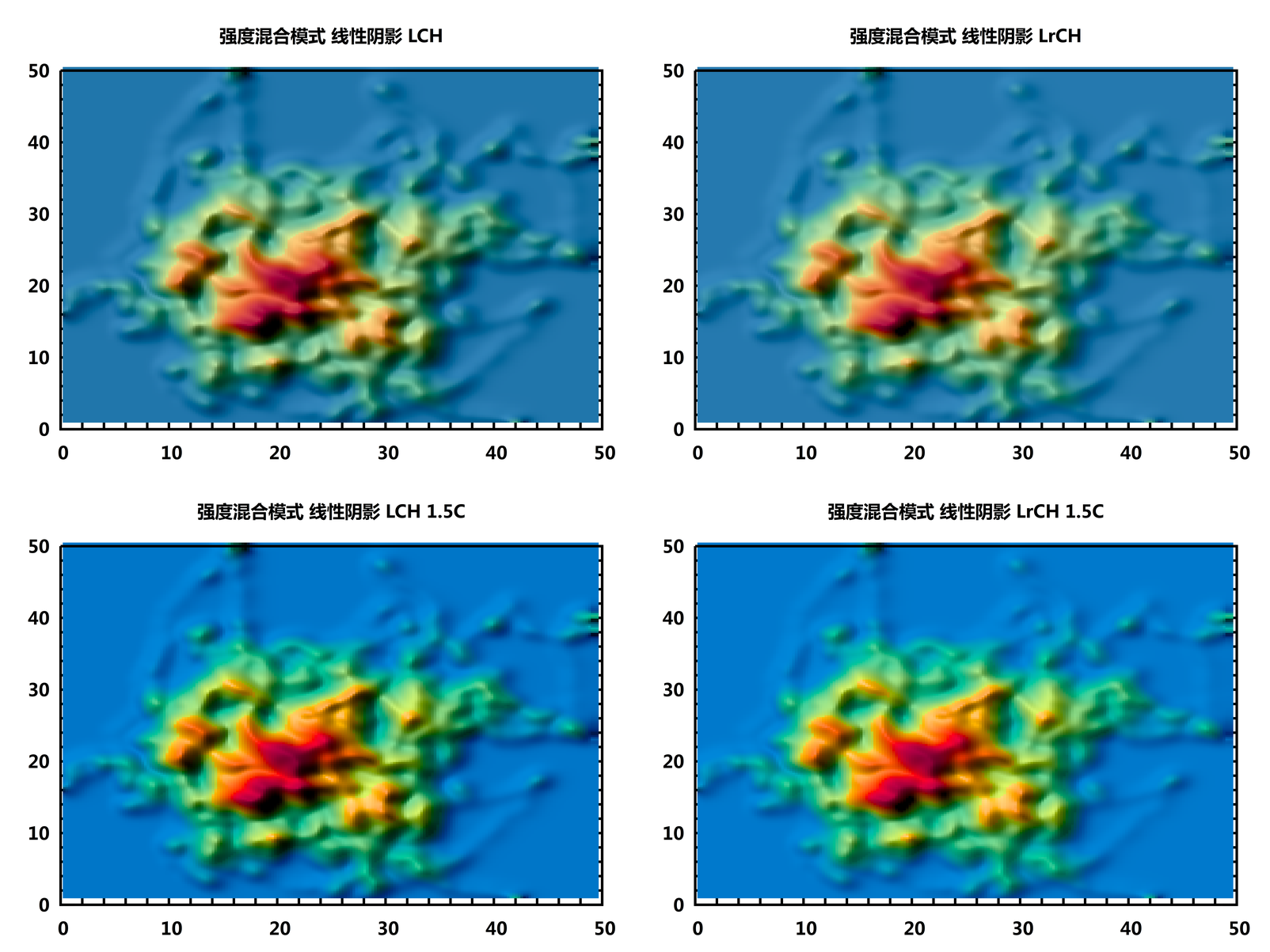# 颜色空间与颜色混合

• 2022-05-20 22:02:46

## 颜色空间

• sRGB: 显示设备所用的标准RGB, 偏硬件
• HSL/HSV/HSB: 调色常用的, 偏设计
• YUV: 电视, 视频用的
• XYZ: 描述人眼对光谱的响应, 偏物理, 一般不会直接用到, 而是作为其他颜色空间的基础
• Lab: 描述人眼对颜色的感知

## 含强度的颜色空间

### YUV颜色空间

YUV颜色空间是一个统称, 有多种不同的变体, 如果名称中使用了Y'则表示颜色值已包含了伽马校正. 根据维基百科的说明, YUV有几种不同的标准, 标准的完整名称类似ITU-R Recommendation BT.2020, 有时会简写为Rec. BT.2020, Rec. 2020, BT.2020等. 在这些标准中, BT.609针对标清视频, BT.709针对HD高清视频, BT.2020针对超高清视频. BT.709的色域和sRGB大致相同, BT.2020的色域则大得多, 但目前用的还比较少, 因为很多显示器还不支持.

BT.470标清

$╤ W_R &= 0.299 \\ W_G &=1-W_R-W_B=0.587 \\ W_B &= 0.114 \\ U_{\max} &= 0.436 \\ V_{\max} &= 0.615 ╧$ $╤ Y' &= W_R R' +W_G G' +W_B B' \\ &= 0.299 R' +0.587 G' +0.114 B' \\ U &= {U_{\max} ／1-W_B} （B'-Y'） \\ &= {U_{\max} ／1-W_B} （B'-W_R R' -W_G G' -W_B B'） \\ &= {U_{\max} ／1-W_B} （-W_R R' -W_G G' +(1-W_B) B'） \\ &= { U_{\max}／1-W_B} （-W_R R' -W_G G'） +U_{\max} B' \\ &= {0.436／0.886}（-0.299 R' -0.587 G'） + 0.436B' \\ &= 0.4920993227990971 ×（-0.299 R' -0.587 G'） + 0.436B' \\ &= -0.14713769751693 R' -0.28886230248307 G' + 0.436B' \\ V &= {V_{\max} ／1-W_R} （R'-Y'） \\ &= {V_{\max} ／1-W_R} （R'-W_R R' -W_G G' -W_B B'） \\ &= {V_{\max} ／1-W_R} （(1-W_R) R' -W_G G' -W_B B'） \\ &= V_{\max} R' + {V_{\max} ／1-W_R} （-W_G G' -W_B B'） \\ &= 0.615 R' +{0.615／0.701}（ -0.587 G'- 0.114B'） \\ &= 0.615 R' +0.877318116975749×（ -0.587 G'- 0.114B'） \\ &= 0.615 R'- 0.5149857346647646 G' - 0.1000142653352354 B' ╧$

BT.610标清

$╤ W_R &= 0.299 \\ W_G &=1-W_R-W_B=0.587 \\ W_B &= 0.114 \\ U_{\max} &= 0.5 \\ V_{\max} &= 0.5 ╧$

BT.709高清

$╤ W_R &= 0.2126 \\ W_G &=1-W_R-W_B=0.7152 \\ W_B &= 0.0722 \\ U_{\max} &= 0.436 \\ V_{\max} &= 0.615 ╧$ $╤ Y' &= W_R R' +W_G G' +W_B B' \\ &= 0.2126 R' +0.7152 G' +0.0722 B' \\ U &= {U_{\max} ／1-W_B} （B'-Y'） \\ &= {U_{\max} ／1-W_B} （B'-W_R R' -W_G G' -W_B B'） \\ &= {U_{\max} ／1-W_B} （-W_R R' -W_G G' +(1-W_B) B'） \\ &= { U_{\max}／1-W_B} （-W_R R' -W_G G'） +U_{\max} B' \\ &= {0.436／0.9278}（-0.2126 R' -0.7152 G'） + 0.436B' \\ &= 0.4699288639793059 ×（-0.2126 R' -0.7152 G'） + 0.436B' \\ &= -0.09990687648200043 R' -0.3360931235179995 G' + 0.436B' \\ V &= {V_{\max} ／1-W_R} （R'-Y'） \\ &= {V_{\max} ／1-W_R} （R'-W_R R' -W_G G' -W_B B'） \\ &= {V_{\max} ／1-W_R} （(1-W_R) R' -W_G G' -W_B B'） \\ &= V_{\max} R' + {V_{\max} ／1-W_R} （-W_G G' -W_B B'） \\ &= 0.615 R' +{0.615／0.7874}（ -0.7152 G' -0.0722 B'） \\ &= 0.615 R' +0.7810515621031242×（-0.7152 G' -0.0722 B'） \\ &= 0.615 R' - 0.5586080772161544 G' - 0.05639192278384557 B' ╧$

BT.2020超高清

$╤ W_R &= 0.2627 \\ W_G &=1-W_R-W_B=0.678 \\ W_B &= 0.0593 \\ U_{\max} &= 0.436 \\ V_{\max} &= 0.615 ╧$

### okLab颜色空间

okLab颜色空间的换算公式, 直接看其作者给出的代码即可. 但具体数字似乎有点小问题, 我没有验证.

## 不同颜色空间混合效果的对比◆本文地址: , 转载请注明◆
◆评论问题: https://jerkwin.herokuapp.com/category/3/博客, 欢迎留言◆访问人次(2015年7月 9日起): | 最后更新: 2022-12-17 03:04:57 UTC | 版权所有 © 2008 - 2022 Jerkwin